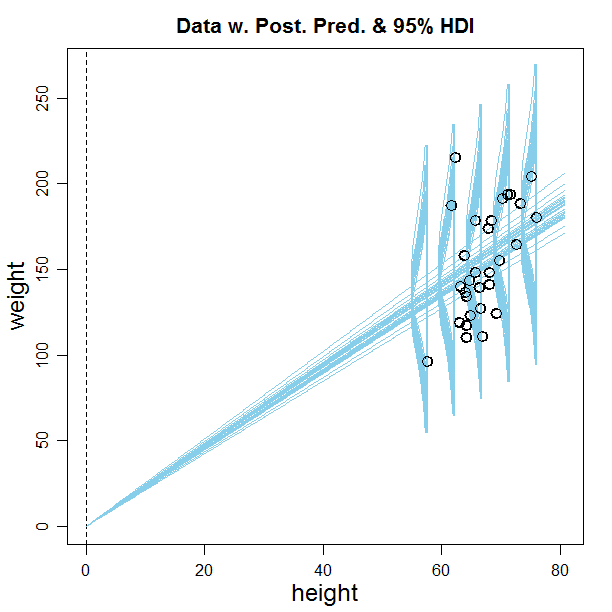## Thursday, June 23, 2016

### Fixing the intercept at zero in Bayesian linear regression

In DBDA2E and in workshops, I present an example of simple linear regression: predicting an adult's weight from his/her height. A participant at a recent workshop suggested that maybe the y-intercept should be fixed at zero, because a person of zero height should have zero weight. I replied that the linear trend is really only intended as a description over the range of reasonable adult heights, not to be extrapolated all the way to a height of zero. Nevertheless, in principle it would be easy to make the restriction in the JAGS model specification. But then it was pointed out to me that the JAGS model specification in DBDA2E standardizes the variables -- to make the MCMC more efficient -- and setting the y intercept of the standardized y to zero is (of course) not the same as setting the y intercept of the raw scale to zero. This blog post shows how to set the y intercept on the raw scale to zero.

For reference, here (below) is the original model specification that does not fix the y intercept at zero. (This is in file Jags-Ymet-Xmet-Mrobust.R.) Notice two lines below in bold font and yellow highlight, regarding the prior on the standardized intercept zbeta0 and the transformation back to the raw-scale intercept beta0:

# Standardize the data:
data {
Ntotal <- length(y)
xm <- mean(x)
ym <- mean(y)
xsd <- sd(x)
ysd <- sd(y)
for ( i in 1:length(y) ) {
zx[i] <- ( x[i] - xm ) / xsd
zy[i] <- ( y[i] - ym ) / ysd
}
}
# Specify the model for standardized data:
model {
for ( i in 1:Ntotal ) {
zy[i] ~ dt( zbeta0 + zbeta1 * zx[i] , 1/zsigma^2 , nu )
}
# Priors vague on standardized scale:
zbeta0 ~ dnorm( 0 , 1/(10)^2 )
zbeta1 ~ dnorm( 0 , 1/(10)^2 )
zsigma ~ dunif( 1.0E-3 , 1.0E+3 )
nu ~ dexp(1/30.0)
# Transform to original scale:
beta1 <- zbeta1 * ysd / xsd
beta0 <- zbeta0 * ysd  + ym - zbeta1 * xm * ysd / xsd
sigma <- zsigma * ysd
}

Now how to fix beta0 to zero: Instead of putting a broad prior distribution on zbeta0, it is set to whatever value would make raw-scale beta0 equal zero. To determine that corresponding value of zbeta0, we use the transform-to-raw-scale formula for beta0, shown at the end of the model specification above. In that formula, set beta0 to 0, then solve for zbeta0. Replace the prior on zbeta0 as follows:

# zbeta0 ~ dnorm( 0 , 1/(10)^2 )
# Fix y intercept at zero:
zbeta0 <- zbeta1*xm/xsd - ym/ysd

When run on the height/weight data (using script Jags-Ymet-Xmet-Mrobust-Example.R), a smattering of regression lines from the posterior distribution looks like this:You can see that the y-intercept has indeed been fixed at zero for all the regression lines.

One crucial trick to getting the graphs to display: In the plotMCMC command of Jags-Ymet-Xmet-Mrobust-Example.R, set showCurve to TRUE:

plotMCMC( mcmcCoda , data=myData , xName=xName , yName=yName ,
compValBeta1=0.0 , ropeBeta1=c(-0.5,0.5) ,
pairsPlot=TRUE , showCurve=TRUE ,
saveName=fileNameRoot , saveType=graphFileType )

If showCurve=FALSE, then it tries to make a histogram which fails for the weird values of beta0 generated by the MCMC.

#### 1 comment:

1.[Thomas Leonard] Maybe a quadratic through zero would be more sensible. With linear regression, the zero would presumably be refuted by the usual t-test.

[John K. Kruschke] Of course, we wouldn't use a frequentist t test! :-)

[Diego Andrés Pérez Ruiz] Do you mean Region of Practical Equivalence (ROPE) ?

[Thomas Leonard] maybe we don't need anything as fancy as that, I'm just eyeballing the data.

[John K. Kruschke] When the intercept is not fixed at zero, Figure 17.3 of DBDA2E shows the estimate of the intercept (see image). The 95% HDI on the estimate includes zero, so we definitely would not want to reject zero. (Image is posted on Facebook here.)

There are two different issues here. One is assessing an intercept of zero. There are two Bayesian approaches to assessing null values; you can read about those in Chapter 12 of DBDA2E, and in this manuscript.

The second issue is what kind of trend curve to use for describing the relation of weight to height for adults, and whether that curve should be constrained to go through the point <0,0>. We might want to use a linear trend to describe the relation for adults, only over the reasonable range of adult heights, withOUT fixing the intercept at zero, because the linear trend is not intended to be extrapolated beyond the reasonable range. In other words, the trend is purely descriptive; the trend is not a process model of growth since the moment of conception. If, on the other hand, one wants a a trend to capture growth over time, that is another issue entirely. Perhaps in that case we would want the data to include age, and the the trend would be some curved function of age and height, and in that case we might fix the intercept at zero.